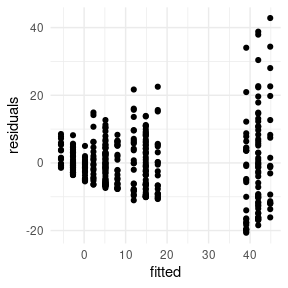# Installation

Batchtma can be installed from GitHub using:

# install.packages("remotes")  # The "remotes" package needs to be installed
remotes::install_github("stopsack/batchtma")

To have vignettes like this current site be available offline via, e.g., vignette("batchtma"), modify the last command:

remotes::install_github("stopsack/batchtma", build_vignettes = TRUE)

# Set up and plot example data

We load the batchtma package and, for convenience with data handling and plotting, the tidyverse package:

library(batchtma)
library(tidyverse)
#> Warning in system("timedatectl", intern = TRUE): running command 'timedatectl'
#> had status 1

We construct a toy dataset of 10 individuals (e.g., tumors), each with 40 measurements (e.g., cores on tissue microarrays) per batch. Unlike perhaps in the real world, for each individual tumor, we also have measurements on all the other batches.

set.seed(123)  # for reproducibility
df <- tibble(
# Batches:
batch = rep(paste0("batch", LETTERS[1:4]), times = 100),
batchnum = rep(c(1, 5, 2, 3), times = 100),
# Participants:
person = rep(letters[1:10], each = 40),
# Instead of a confounder, we will use a random variable for now:
random = runif(n = 400, min = -2, max = 2),
# The true (usually unobservable biomarker value):
true = rep(c(2, 2.5, 3, 5, 6, 8, 10, 12, 15, 12), each = 40),
# The observed biomarker value with random error ("noise"):
noisy = true + runif(max = true / 3, n = 400) * 4)

df
#> # A tibble: 400 x 6
#>    batch  batchnum person random  true noisy
#>    <chr>     <dbl> <chr>   <dbl> <dbl> <dbl>
#>  1 batchA        1 a      -0.850     2  4.63
#>  2 batchB        5 a       1.15      2  2.37
#>  3 batchC        2 a      -0.364     2  4.41
#>  4 batchD        3 a       1.53      2  3.54
#>  5 batchA        1 a       1.76      2  3.05
#>  6 batchB        5 a      -1.82      2  3.20
#>  7 batchC        2 a       0.112     2  3.88
#>  8 batchD        3 a       1.57      2  2.22
#>  9 batchA        1 a       0.206     2  2.90
#> 10 batchB        5 a      -0.174     2  3.82
#> # … with 390 more rows

We plot the biomarker values (y-axis) by batch (x-axis), using the plot_batch() function. Color/shape symbolizes which participant/tumor the measurements came from. Boxes span from the 25th to the 75th percentile (interquartile range); thick lines indicate medians; asterisks indicate means.

df %>% plot_batch(marker = noisy, batch = batch, color = person)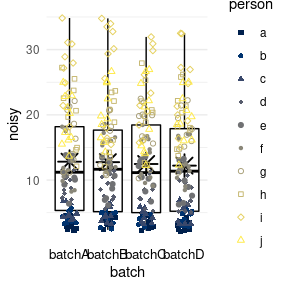## Add batch effects

We add systematic differences between batches such that there is differential measurement error between batches in terms of mean and variance. As shown above, true values were the same beyond random error.

df <- df %>%
# Multiply by batch number to differentially change variance by batch,
# divide by mean batch number to keep overall variance the same:
mutate(noisy_batch = noisy * batchnum / mean(batchnum) +
# Similarly, change mean value per batch, keeping overall mean the same:
batchnum * 3 - mean(batchnum) * 3)

df %>% plot_batch(marker = noisy_batch, batch = batch, color = person)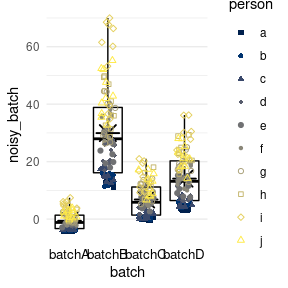# Perform batch effect adjustment without confounders

## Simple means

method = simple calculates the mean for each batch and subtracts the difference between this mean and the grand mean, such that all batches end up having a mean equivalent to the grand mean. Differences in variance between batches will remain, if they exist (as in this example).

df %>%
adjust_batch(markers = noisy_batch, batch = batch,
method = simple) %>%
plot_batch(marker = noisy_batch_adj2, batch = batch, color = person)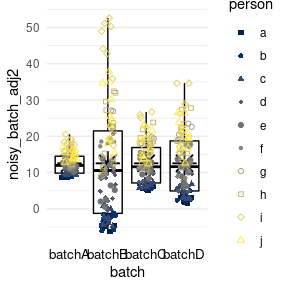## Means from marginal standardization

method = standardize performs marginal standardization by fitting a linear regression model for biomarker values with batch and confounders as predictors, and obtains the marginal means per batch if they had the same distribution of confounders. Differences between these marginal means and the grand mean are subtracted as in method = simple. In this example, the confounder is a random variable, and the results are essentially the same as for method = simple.

df %>%
adjust_batch(markers = noisy_batch, batch = batch,
method = standardize, confounders = random) %>%
plot_batch(marker = noisy_batch_adj3, batch = batch, color = person)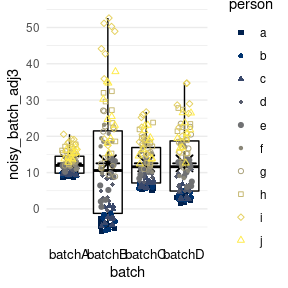## Inverse-probability weighting

method = ipw predicts the probability of a measurement being from a specific batch, given the confounders. Mean differences between batches are obtained from a marginal structural model with stabilized inverse-probability weights and then used as in the two preceding methods. Again, the confounder is merely a random variable in this example.

df %>%
adjust_batch(markers = noisy_batch, batch = batch,
method = ipw, confounders = random) %>%
plot_batch(marker = noisy_batch_adj4, batch = batch, color = person)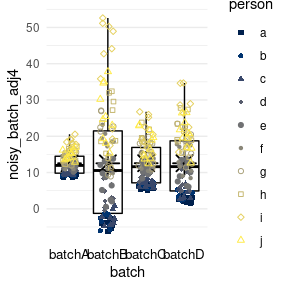## Quantile regression

method = quantreg, unlike the three preceding mean-only methods, addresses two distinct properties of batches: the offset values (a lower quantile), potentially reflective of background signal, and an inter-quantile range, potentially reflective of the dynamic range of the measurement. By default, the first and third quartile are used.

df %>%
adjust_batch(markers = noisy_batch, batch = batch,
method = quantreg, confounders = random) %>%
plot_batch(marker = noisy_batch_adj5, batch = batch, color = person)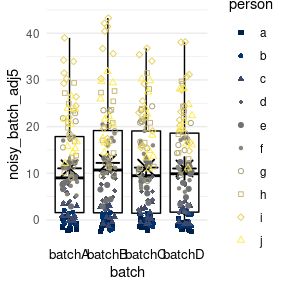## Quantile normalization

method = quantnorm performs quantile normalization: values are ranked within each batch, and then each rank is assigned the mean per rank across batches. Quantile normalization ensures that all batches have near-identical biomarker distributions. However, quantile normalization does not allow for accounting for confounders.

df %>%
adjust_batch(markers = noisy_batch, batch = batch,
method = quantnorm) %>%
plot_batch(marker = noisy_batch_adj6, batch = batch, color = person)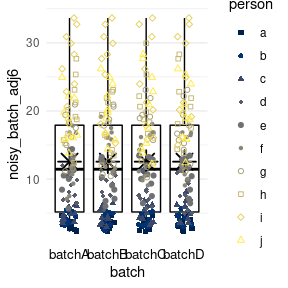# Perform batch effect adjustment in the presence of confounding

## Set up example data with confounding present

In general, confounding means that exposure and outcome share common causes. Necessary—but not sufficient—properties of a confounder are that it needs to be associated with the exposure (batch) and associated with the outcome(s) (markers). For example, if systematically higher biomarker values due to batch effects occur in a tissue microarray with a higher proportion of aggressive tumors, then batch effect-adjusted biomarker values should account for the differences in proportions of aggressive tumors.

In the example, a new variable for the biomarker is generated that is affected by both confounding and batch effects. First, biomarker values with random error, noisy, are forced to be associated with the confounder, which in turn is associated with batch. These values, noisy_conf, should be considered the new “ground truth.”

All following plots show color/symbol shape by confounder.

set.seed(123)  # for reproducibility
df <- df %>%
# Make confounder associated with batch:
mutate(confounder = round(batchnum + runif(n = 200, max = 2)),
# Make biomarker values associated with confounder:
noisy_conf = noisy + confounder * 3 - mean(confounder) * 3)

df %>% plot_batch(marker = noisy_conf, batch = batch, color = confounder)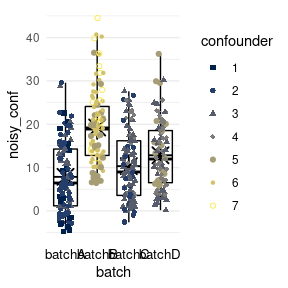Second, batch effects for mean and variance are added.

df <- df %>%
# Add batch effects to confounded biomarker values:
mutate(noisy_conf_batch = noisy_conf * batchnum / mean(batchnum) +
batchnum * 3 - mean(batchnum) * 3)

df %>% plot_batch(marker = noisy_conf_batch, batch = batch, color = confounder)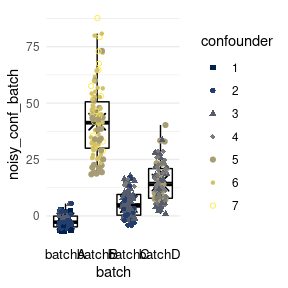The following examples show to what extent batch effects can be removed from noisy_conf_batch to recover the “ground truth,” noisy_conf.

## Means from marginal standardization

method = standardize reduces batch effects while allowing batch means to differ because of confounders. Batches with higher true biomarker values associated with the confounders, like batch B, retain higher means after batch effect adjustment.

df %>%
adjust_batch(markers = noisy_conf_batch, batch = batch,
method = standardize, confounders = confounder) %>%
plot_batch(marker = noisy_conf_batch_adj3, batch = batch, color = confounder)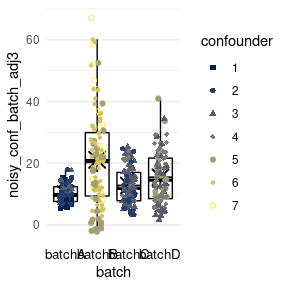## Inverse-probability weighting

method = ipw also reduces batch effects while allowing batch means to differ because of confounders. As with marginal standardization, differences in variance between batches are not addressed.

df %>%
adjust_batch(markers = noisy_conf_batch, batch = batch,
method = ipw, confounders = confounder) %>%
plot_batch(marker = noisy_conf_batch_adj4, batch = batch, color = confounder)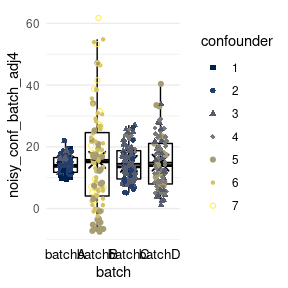## Quantile regression

method = quantreg reduces for batch effects of offset and dynamic range separately, accounting for differences in confounders. In the example, batch B with its high levels of the confounder retains somewhat higher values and a higher variance, unlike with the two preceding mean-only methods.

df %>%
adjust_batch(markers = noisy_conf_batch, batch = batch,
method = quantreg, confounders = confounder) %>%
plot_batch(marker = noisy_conf_batch_adj5, batch = batch, color = confounder)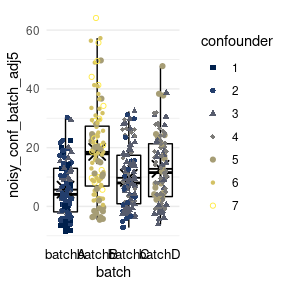## For comparison: Batch effect adjustment ignoring confounding

### Simple means

method = simple leads to all batches having the same mean after adjustment. In the example, this method ignores that batch B should have higher values because of higher values of the confounder.

df %>%
adjust_batch(markers = noisy_conf_batch, batch = batch,
method = simple, confounders = confounder) %>%
plot_batch(marker = noisy_conf_batch_adj2, batch = batch, color = confounder)
#> Batch effect correction via 'method = simple' was requested.
#>  This method does not support adjustment for confounders (confounder). They will be ignored.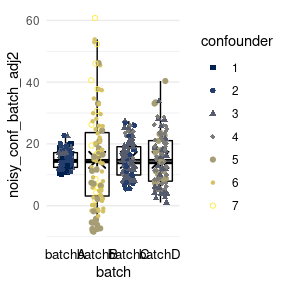### Quantile regression

method = quantreg also ignores the confounder.

df %>%
adjust_batch(markers = noisy_conf_batch, batch = batch,
method = quantnorm, confounders = confounder) %>%
plot_batch(marker = noisy_conf_batch_adj6, batch = batch, color = confounder)
#> Batch effect correction via 'method = quantnorm' was requested.
#>  This method does not support adjustment for confounders (confounder). They will be ignored.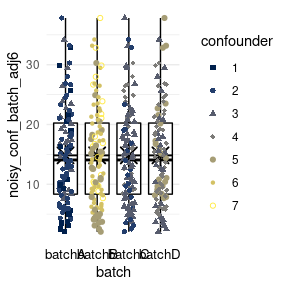## Missing values

batchtma can handle missing biomarker values, which is a common phenomenon in tissue microarray studies. No coding changes are needed.

This situation is different from gene expression studies, where measured genes are typically measured on all batches (e.g., microarrays) and methods like ComBat can “borrow” information from the other markers (genes) measured on the same batch.

In batchtma, adjustments are done separately for each marker, even if multiple biomarkers are batch-adjusted with one call of adjust_batch(markers = c(biomarker_a, biomarker_b, biomarker_c), ...) and different rows (e.g., tumors) have missing values for different columns (biomarkers). Thus, no values will be excluded because of missingness in another marker.

## Model diagnostics

When running adjust_batch(), the dataset is returned with the batch effect-adjusted variables added at the end. Yet what did adjust_batch() do and how well did the methods used for batch effect correction fit to the data that they were applied to? Adjustment for batch effects relies on regression models for methods standardize, ipw, and quantreg; in addition, method = simple estimates means per batch.

The diagnose_models() function provides an overview of what adjust_batch() did, shows overview of the adjustment models, and provides access to detailed model diagnostics.

To obtain an overview of model diagnostics, diagnose_models() is called on the return values of adjust_batch():

# df2 is the new dataset that also contains "noisy_conf_batch_adj2":
df2 <- df %>% adjust_batch(markers = noisy_conf_batch, batch = batch,
method = standardize, confounders = confounder)

# Show overview of model diagnostics:
diagnose_models(df2)
#> Dataset after batch effect adjustment using 'method = standardize'
#> Variable defining batches: batch
#> Confounders: confounder
#>
#> Estimated adjustment parameters:
#> # A tibble: 4 x 3
#>   marker           .batchvar batchmean
#>   <chr>            <chr>         <dbl>
#> 1 noisy_conf_batch batchA      -12.4
#> 2 noisy_conf_batch batchB       20.6
#> 3 noisy_conf_batch batchC       -7.51
#> 4 noisy_conf_batch batchD       -0.683
#>
#> Models for adjustment:
#> []
#> [][]
#>
#> Call:
#> stats::lm(formula = stats::as.formula(paste0("value ~ .batchvar +",
#>     paste(confounders, collapse = " + ", sep = " + "))), data = .x)
#>
#> Coefficients:
#>     (Intercept)  .batchvarbatchB  .batchvarbatchC  .batchvarbatchD
#>          -8.469           33.079            4.930           11.759
#>      confounder
#>           2.893

In this example, method = standardize fits a linear regression model with batch and confounders as exposure and the unadjusted biomarker as the outcome. This model can be extracted directly from diagnose_models():

fit <- diagnose_models(data = df2)$model_fits[][] summary(fit) #> #> Call: #> stats::lm(formula = stats::as.formula(paste0("value ~ .batchvar +", #> paste(confounders, collapse = " + ", sep = " + "))), data = .x) #> #> Residuals: #> Min 1Q Median 3Q Max #> -20.634 -5.834 -0.714 4.626 42.827 #> #> Coefficients: #> Estimate Std. Error t value Pr(>|t|) #> (Intercept) -8.4686 1.7092 -4.955 1.08e-06 *** #> .batchvarbatchB 33.0786 2.9225 11.319 < 2e-16 *** #> .batchvarbatchC 4.9297 1.4606 3.375 0.000811 *** #> .batchvarbatchD 11.7585 1.8269 6.436 3.55e-10 *** #> confounder 2.8926 0.6837 4.231 2.90e-05 *** #> --- #> Signif. codes: 0 '***' 0.001 '**' 0.01 '*' 0.05 '.' 0.1 ' ' 1 #> #> Residual standard error: 9.275 on 395 degrees of freedom #> Multiple R-squared: 0.7684, Adjusted R-squared: 0.7661 #> F-statistic: 327.7 on 4 and 395 DF, p-value: < 2.2e-16 The marginally standardized means per batch are the adjustment parameters estimated by this procedure. They are returned by diagnose_models() as adjust_parameters: diagnose_models(df2)$adjust_parameters
#> # A tibble: 4 x 3
#>   marker           .batchvar batchmean
#>   <chr>            <chr>         <dbl>
#> 1 noisy_conf_batch batchA      -12.4
#> 2 noisy_conf_batch batchB       20.6
#> 3 noisy_conf_batch batchC       -7.51
#> 4 noisy_conf_batch batchD       -0.683

Diagnostics for regression models can be performed on fitted models just as for any other regression model in R. For example, residuals vs. fitted can be plotted:

tibble(fitted    = fitted.values(fit),
residuals = residuals(fit)) %>%
ggplot(mapping = aes(x = fitted, y = residuals)) +
geom_point() +
theme_minimal()